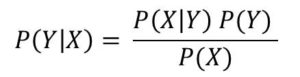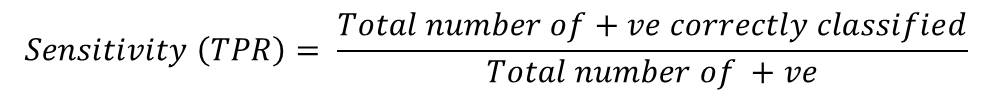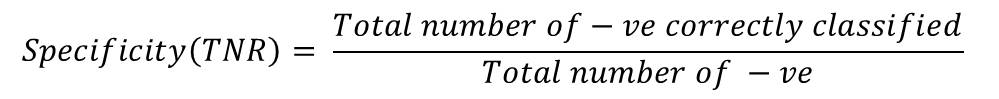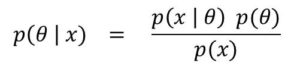# Machine Learning Questions and Answers – (Question 1 to 10)

I’m happy to the making this lesson. I would give you brief answers to several Machine Learning questions. But if you would like to go in-depth, then you can watch the video explanation of the answers.
You can find Question 1 to 20 below

Question 11 to 20.
So let’s get started!

##### 1. What is Maximum Likelihood Estimation(MLE)?

Maximum Likelihood Estimation is a procedure used to estimate an unknown parameter of a model. MLE  is based on the Likelihood Function and it works by making an estimate the maximizes the likelihood function.  The likelihood function is simply a function of the unknown parameter, given the observations(or sample values).

Therefore, maximum likelihood estimate is the value of the parameter that maximizes the likelihood of getting the the observed data.

##### 2. Explain Decision Theory in Machine Learning?

First, I would like to remind you that the three fundamental theories of machine learning are

Probability Theory, Information Theory and Decision Theory.

Now, decision theory  in Machine Learning is the strategies and method involved in choosing a particular action among a number of probable actions.

##### 3. What is Bayesian Model

Bayesian Model is a probabilistic model (a system of making inference) that is based on Bayes’ Theorem. The Bayesian model attempts to obtain a  posterior distribution base on some prior distribution.

For example, if we have the density function for some observations Xi for i = 1 to n to be f(Xi | θ) for unknown parameter θ.

Then the prior distribution is given by p(θ), Bayesian model would try to find the parameter using the posterior p(θ | X)

Just as a reminder, you can find Bayes’ Theorem below:I recommend you watch the video explanation. You’ll understand it better.

##### 4. Differentiate between Sensitivity and Specificity

First, I would like to mention that this two terms are related to classification. They are used to describe the performance of a binary classifier.

Sensitivity is the same as true positive rate(TPR): It provides a measure of actual positives that were classified correctly. Formula for sensitivity is given as:Specificity is the same as true negative rate(TNR): It measures the actual negatives that were correctly classified against the total number of negatives. Formula for specificity is given below:Illustration of Sensitivity and Specificity: Assuming you build a binary classifier to predict if patients have cancer. The classifier, outputs 1 if it thinks patient have cancer and 0 if otherwise. If 100 patients are examined, and the classifier, predicts 25 patient’s as having cancer whereas the real number of patients having cancer 28, then the Sensitivity would be 25/28 = 0.89.

You can do the same calculation for Specificity.

• ##### Likelihood

All these terms follows from the Bayes’ Theorem. In fact these are all you get when you state Bayes’ Theorem.

So if we are trying to deduce the distribution for a parameter θ,  given some set of observation x, then we can obtain the posterior distribution using Bayes’ Rule as follows:From the formula above, the term p(θ |x) is known as the posterior probability while the term p(θ) is known as the prior probability. Also, the term p(x | θ) is known as the likelihood. Now, if  the posterior and the prior are in the same probability distribution family, then they are referred to as conjugate prior and conjugate posterior.

Question 5 Video Explanation

##### 6. What is Dimensionality Reduction

Dimensionality reduction is a procedure used to a dataset with large number of variables using a few principal variables. These can be done by either feature selection or feature extraction. In feature selection, we try to find a subset of the original features while in feature extraction we transform the original data to obtain a new set of features.

##### 7. What is Feature Selection

Feature Selection is a dimensionality reduction technique used to transform a dataset from a high-dimensional space to fewer dimension. In feature extraction, the data is represented in a completely new dimension fewer than the original dimension.

##### 8. Briefly Explain Principal Components Analysis (PCA)

PCA is a dimensionality reduction technique that makes use of feature extraction. PCA is a procedure that applies orthogonal transformation to transform a set of data of correlated features into dataset of values of linearly uncorrelated variables known as principal components.

##### 9. What is Eigen-value Plot

This is also known as graph of eigen-values.

##### 10. What is a Biplot

Biplots are a kind of two-variable scatterplot that allows information on both a data matrix and a sample to be displayed on a graph#### kindsonthegenius

Kindson Munonye is currently completing his doctoral program in Software Engineering in Budapest University of Technology and Economics

View all posts by kindsonthegenius →